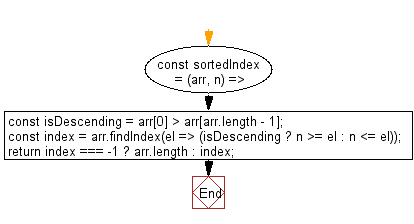# JavaScript: Get the lowest index at which value should be inserted into array in order to maintain its sort order

## JavaScript fundamental (ES6 Syntax): Exercise-142 with Solution

Write a JavaScript program to get the lowest index at which values should be inserted into an array in order to maintain its sorting order.

Note: Check if the array is sorted in descending order (loosely).

• Loosely check if the array is sorted in descending order.
• Use Array.prototype.findIndex() to find the appropriate index where the element should be inserted.

Sample Solution:

JavaScript Code:

``````//#Source https://bit.ly/2neWfJ2
const sortedIndex = (arr, n) => {
const isDescending = arr > arr[arr.length - 1];
const index = arr.findIndex(el => (isDescending ? n >= el : n <= el));
return index === -1 ? arr.length : index;
};

console.log(sortedIndex([5, 3, 2, 1], 4));
console.log(sortedIndex([30, 50], 40));
```
```

Sample Output:

```1
1
```

Flowchart:Live Demo:

See the Pen javascript-basic-exercise-142-1 by w3resource (@w3resource) on CodePen.

Improve this sample solution and post your code through Disqus

What is the difficulty level of this exercise?

Test your Programming skills with w3resource's quiz.

﻿